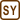## Satellite Altitude

Determine at what altitude above the equator will the orbit stationary satellite flow.(It is also called fixed satellite, because its position is fixed above the same place on Earth’s surface).

Solve the task in the inertial and non-inertial frame of reference.

• #### Notation

 T = 24 h Time for one rotation of the Earth about its axis

From a physical tables:

 G = 6.67·10−11 N m2 kg−2 Gravitational constant M = 5,97·1024 kg The mass of the Earth R = 6378 km The radius of the Earth
• #### Hint 1 – Solution in the Inertial Frame of Reference

Think about the forces acting on the satellite and their effects.

• #### Hint 2

Write down the Newton’s law of gravitation and the formula for centripetal force.

• #### Hint 3

Think over the satellite’s velocity, to make it geostationary.

• #### Hint 4

By using formulas (1) and (2) express the unknown height.

• #### Hint 5 – Solution in the Non-Inertial Frame of Reference

Think about the forces acting on the satellite and their effects.

• #### Complete Solution

Task will be observed from the perspective of the inertial frame of reference

The satellite is moving in a uniform circular motion. Only the force acting on it is a gravitational force, acting also as a centripetal force and curve the satellite trajectory. Thus the gravitational force and the centripetal force are equal.

Write down the Newton’s law of gravitation and the formula for centripetal force.

Newton’s law of gravitation:

$F_{g} = G\frac{mM}{\left( R+h\right)^{2}}\, ,$

where m represents the mass of the satellite and h is the altitude of the satellite above the Earth’s surface.

Centripetal force is equal to:

$F_{c} = ma_d = m\frac{v^{2}}{R+h},$

where v is the peripheral speed of the satellite.

Both of those forces must be equal, thus:

$G\frac{mM}{\left( R+h\right)^{2}} = m\frac{v^{2}}{R+h}\tag{(1)}$

The satellite is a geostationary satellite, thus its angular velocity is similar to the angular velocity of the Earth. Hence the period of this movement is also the same – 24 hours.

Thus the peripheral speed of the satellite is

$v = \omega \left( R+h \right) = \frac{2\pi}{T}\left( R+h \right) ,\tag{(2)}$

where T represents its period.

By using formulas (1) and (2) express the unknown height.

Angular velocity in formula (2) is substituted for v in formula (1).

$G\frac{mM}{\left( R+h \right)^{2}} = \frac{4\pi^{2}m\left( R+h\right)}{T^{2}}\, \Rightarrow \, G\frac{ MT^{2}}{4\pi^{2}} = \left( R+h \right)^{3}$

The final solution for h is:

$h = \sqrt{G\frac{MT^{2}}{4\pi^{2}}}-R\,$

In the perspective of the non-inertial frame of reference which rotates alongside with the satellite, the satellite stays still. Forces acting on it are the gravitational force and the centrifugal force. The resultant of these forces is equal to zero.

$\vec{F}_{g}\,+\,\vec{F}_{c}\,=\,\vec{o}$

Magnitude of the force is

$F_{g} - F_{c} = 0$

thus

$F_{g} = F_{c}$ $F_{g} = G\frac{mM}{\left( R+h\right)^{2}}\, ,$

Magnitude of the centrifugal force is the same as the centripetal force.

$F_{c} = m\frac{v^{2}}{R+h},$

Hence

$G\frac{mM}{\left( R+h\right)^{2}} = m\frac{v^{2}}{R+h}$

The next steps of the solution the same as the previous part.

• #### Numerical Solution

$h = \sqrt{G\frac{MT^{2}}{4\pi^{2}}}-R = (\sqrt{6.67{\cdot}10^{-11}\,\cdot\,\frac{5.97{\cdot}10^{24}\,\cdot\, \left( 86.4{\cdot}10^{3} \right) ^{2}}{4\pi^{2}}}-$ $-6378{\cdot}10^{3}) \,\mathrm{m} \dot{=} 36{\cdot}10^{6}\,\mathrm{m} = 36\, 000\,\mathrm{km}$
Altitude of the satellite: $h\,=\,\sqrt{G\frac{MT^{2}}{4\pi^{2}}}-R$ .